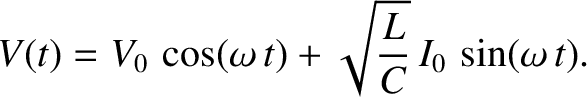# How to create a damped harmonic oscillation?

Hello,

I am building an iPad app and a cube of scale 4 * 1 * 4 and the game is a top down view game. As the user, tilts the iPad I am trying to yield a Damped Harmonic Oscillation on the cube. Hence the oscillation would only be on 2 directions which is X & Z. In layman terms, there would be a wobble on the cube.

GameView:
[3942-screen+shot+2012-10-05+at+10.56.24+am.png|3942]

The game is face negative Y axis or mathematically the camera is tilted 90 degrees across x axis.

Dissecting :

Considering just one direction, the graph would be like this -So if a user gives a input, I would calculate the target value and then apply it to a function which would calculate the required value and then oscillate the cube. I visualize to perform the oscillation by AddForceAtPosition on Vector3.down.

Can you devs help me with this?

regards,

Karsnen

http://phrogz.net/damped-spring-oscillations-in-javascript

http://wiki.unity3d.com/index.php/File:Mathfx-Bounce.png

Hiya, so the graph you want is a SIN or COS wave, so you take time.Time, use it as a ramp, multiply time.Time by a multiplier number to get the speed you want on the sine oscillations, and then multpiply the sine itself by an envelope, in your case it is abit like an exponentially dampening/waning envelope… you can make the waning envelope with some cheap exponential functions from the net, for example one way of making an curved decrease like that, is by taking a ramp (using a version of time.Time that resets to zero every time you want the envelope to retrigger) and doing a sqrt or a cube root or a square or a cube, like this :

if you decide that sin and cos arent exactly right, you can pass cos through asin or sin through acos to have a triangle oscillation, or you can do a modulo of time.time and then make an absolute of that modulo which will also give you a triangle wave and is less processor hungry than sin. you can also multiply that triangle wave by itself to make it rounder.